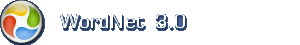# Engelsk ordbog

Tip: Stjerne (*) kan anvendes som jokertegn (wild card). Stjernen erstatter nul eller flere tegn.

## Engelsk navneord: regression coefficient

1. regression coefficient (om erkendelse) when the regression line is linear (y = ax + b) the regression coefficient is the constant (a) that represents the rate of change of one variable (y) as a function of changes in the other (x); it is the slope of the regression line

Mindre specifikke termerparametric statistic

Omfatter disse overordnede termerlinear regression, rectilinear regression

Overordnet emneområdestatistics

Baseret på WordNet 3.0 copyright © Princeton University.
Teknik og design: Orcapia v/Per Bang. Dansk bearbejdning: .
2019 onlineordbog.dk